output.to from Sideway
Mechanics: Statics

Applications of Friction

Draft for Information Only

# Content

``` Applications of Friction Forces  Wedges  Screws```

# Applications of Friction Forces

Frictional forces are found in many common practical engineering tools and machines.

## Wedges

Wedge is a simple tool commonly used in mechanical applications such as mechanical stopper, mechanical splitter or  mechanical lifting device. A wedge is a tool of triangular in shape with one or two inclined planes. Because of the triangular shape of a wedge, a wedge usually raises a load small apart by travelling a longer distance. The applied sideways force for raising the load is also considerably smaller. And a wedge with proper inclined angle after being forced will remain in place under the load due to the friction between surfaces in contant.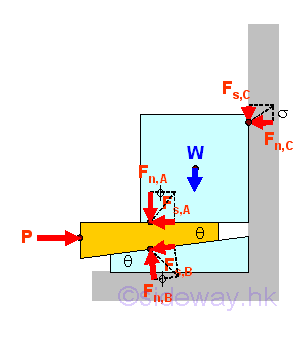When the force P is applied to the wedge, the block of weight W will be raised if the applied force is only enough to cause the wedge about to move forward. The free body diagram of the block is.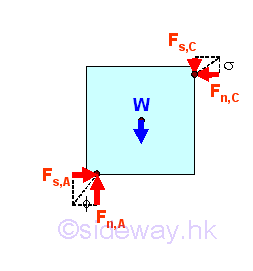Therefore the friction force Fs,A due to the contact surfaces between the block and the wedge will act as the horizontal holding force of the block against the wall and the friction force Fs,A will be balanced by normal reaction force Fn,C . The normal reaction force Fn,C will in turn generate another friction force  Fs,C due to the contact surfaces between the block and the wall. The vertical friction force  Fs,C and the weight W of the block will be supported by the vertical reaction force Fn,A of the wedge. Since the motion of block is impending, Imply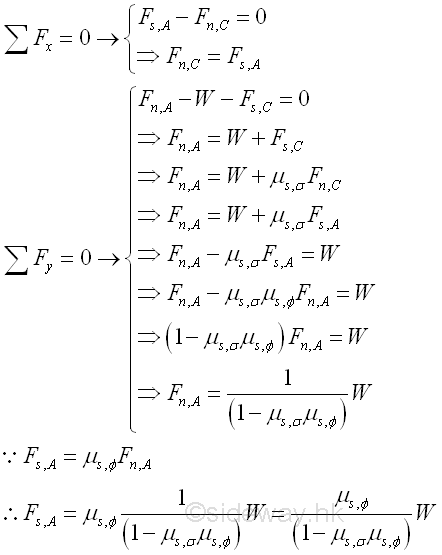Similarly, when the applied force is only enough to cause the wedge about to move forward, the motion wedge is impending also. The free body diagram of the wedge is.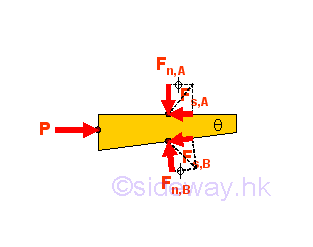Therefore the applied force vector P should be balanced by the vector sum of the resultant force vector FA due to the block and the resultant force vector FB due to the wedge base. Since the wedge has a sloped surface, the equilibrium equations are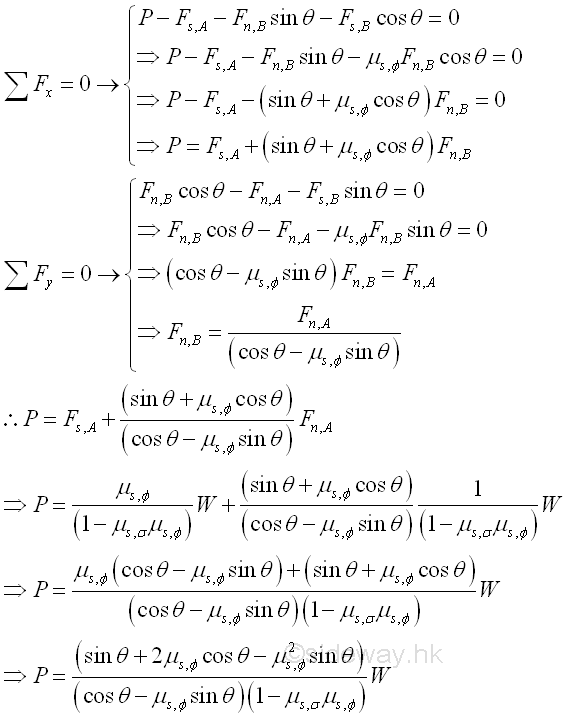## Screws

Screws are common component parts found in mechanical mechanism. For example square-threaded screws and nuts are formed by cutting an external square thread on the external surface of a cylinder and an internal square thread on the internal surface of a hollow cylinder.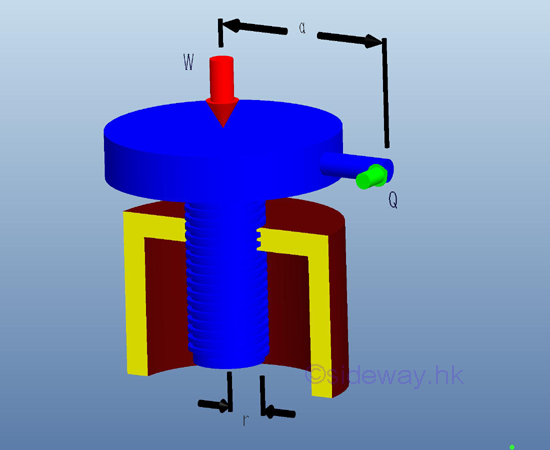In general, screw thread is a helical structure with uniform cross section wrapped around a cylinder. The profile of the helix structure can be formed by wrapping a right angle triangle arround a cylinder. The hypotenuse of the right angle triangle is a straight line and is equal to the length of the helix curve of the screw. The slope of the right angle triangle can be determined by adjacent side  and opposite side. The angle of elevation is equal to the the ratio of base to height. When the base of the triangle is assumed to be equal to the mean circumference of the screw, i.e. 2πr, the height of triangle is equal to the lead L of the screw. The angle of the line of helix structure formed with the horizontal is called lead angle.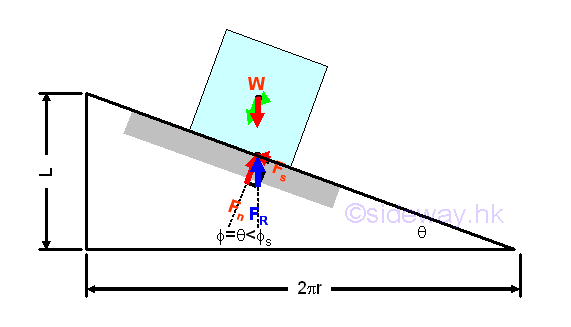Lead is the axial travelled distance of the screw in one revolution of a screw. The definition of pitch is not the same as the lead. Pitch is only the distance of two consecutive threads of a screw. For a screw with one single thread, there is only one screw thread in one revolution of the screw, therefore the axial travelled distance of the screw advances is equal to the pitch  in one revolution of a screw. A single threaded screw has only one single start on the transverse section of the screw. There are also multiple-threaded screws, e.g. double threads with two starts and triple threads with three starts. For double-threaded screw, there are two independent screw threads on the screw or two independent screw threads in one revolution of screw, therefore the pitch is only a half of the lead. For triple-threaded screw, there are three independent screw threads on the screw or three independent screw threads in one revolution of screw, therefore the pitch is only one third of the lead.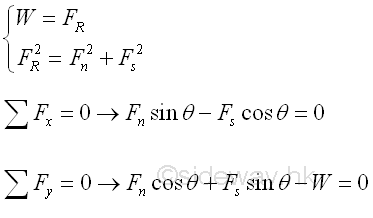As the helix structure of a screw can be represented by an inclined plane, the force anlysis for a screw is similar to the analysis of a sliding block on an inclined plane. In static equilibrium, the load W is balanced by the reaction force FR. When a force Q is exerted on the handle, an equivalent effect will act on the screw thread also. The force P acting on the screw thread can be determined by P=Qa/r. As in sliding block on an inclined board, the friction force for impending motion and the friction force for maintain motion are depending on the coefficient of static friction and coefficient of kinetic friction respectively. Imply

When the applied force P is only enough to cause the load about to move the load upward, the motion of the load is impending. Imply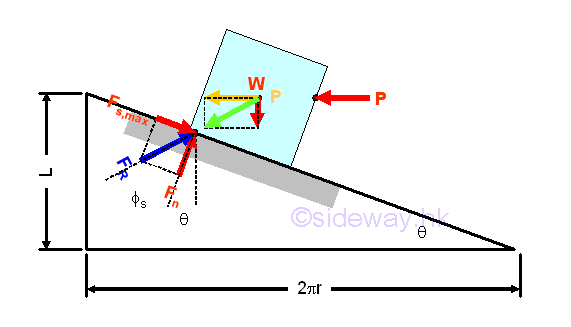The resultant force of weight W and applied force P is balanced by the reaction force FR due to the maximum static friction force Fs,max and the normal reaction force Fn. Imply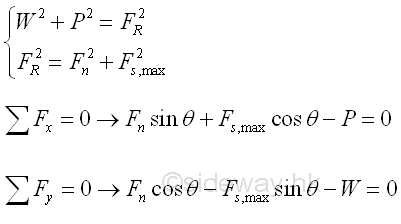When the applied force P is only enough to cause the load about to move the load downward, the motion of the load is impending. Imply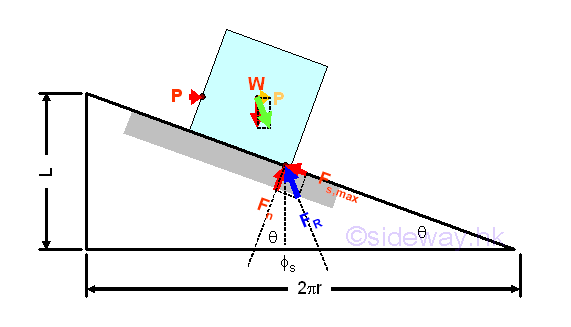The resultant force of weight W and applied force P is balanced by the reaction force FR due to the maximum static friction force Fs,max and the normal reaction force Fn. Imply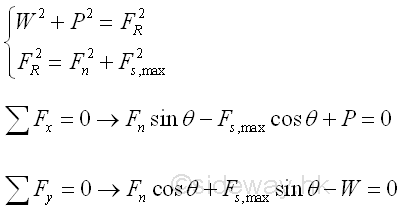Since the angle of friction Φs is greater than the lead angle θ. applied force P is alway needed to unlock the screw, the screw is said to be self-locking. If the applied force P is equal to zero, the block will remain in place under the weight W.

But if the angle of friction Φs is smaller than the lead angle θ, an additional applied force P is needed to maintain the block in place under the weight W. Imply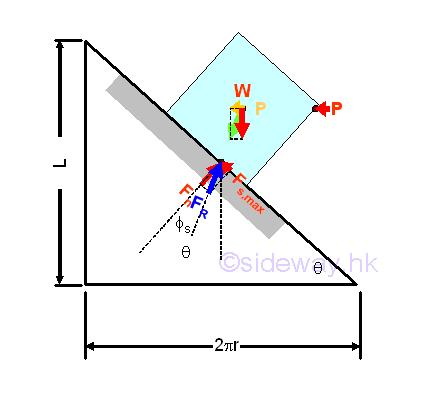The resultant force of weight W and applied force P is balanced by the reaction force FR due to the maximum static friction force Fs,max and the normal reaction force Fn. Imply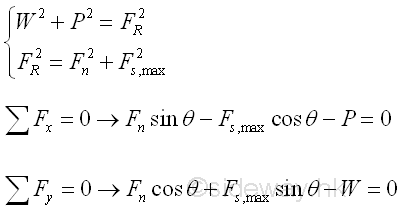Considering forces along the inclined plane. If there is no applied force P,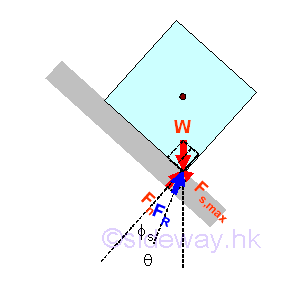The net force along the inclined plane is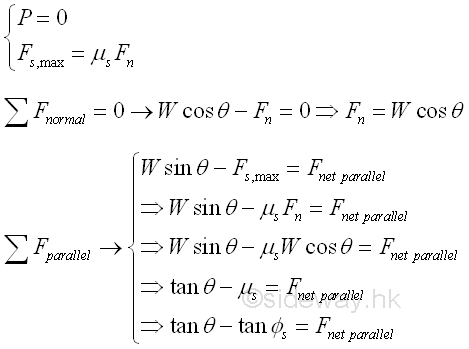Therefore when the angle of friction Φs is smaller than the lead angle θ, the net force parallel to the inclined plane is not equal to zero and forced the block to slide down along the sloped plane. And as a result, the block will unwind the screw under the weight W.

©sidewayReferences

1. I.C. Jong; B.G. rogers, 1991, Engineering Mechanics: Statics and Dynamics, Saunders College Publishing, United States of America
2. F.P. Beer; E.R. Johnston,Jr.; E.R. Eisenberg, 2004, Vector Mechanics for Engineers: Statics, McGraw-Hill Companies, Inc., New YorkID: 120800003 Last Updated: 2012/8/4 Revision: 0 Ref:Home (5)

Business

Management

HBR (3)

Information

Recreation

Hobbies (7)

Culture

Chinese (1097)

English (336)

Reference (66)

Computer

Hardware (149)

Software

Application (187)

Digitization (24)

Numeric (19)

Programming

Web (648)CSS (SC)

ASP.NET (SC)

HTML

Knowledge Base

Common Color (SC)

Html 401 Special (SC)

OS (389)

MS Windows

Windows10 (SC)

.NET Framework (SC)

DeskTop (7)

Knowledge

Mathematics

Formulas (8)

Number Theory (206)

Algebra (20)

Trigonometry (18)

Geometry (18)

Calculus (67)

Complex Analysis (21)

Engineering

Tables (8)

Mechanical

Mechanics (1)

Rigid Bodies

Statics (92)

Dynamics (37)

Fluid (5)

Control

Acoustics (19)

Biology (1)

Geography (1)

Copyright © 2000-2019 Sideway . All rights reserved Disclaimers last modified on 10 Feb 2019